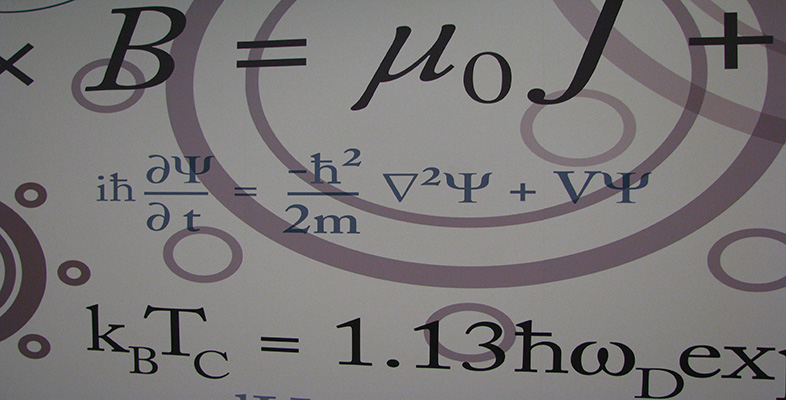Number systems

This free course is available to start right now. Review the full course description and key learning outcomes and create an account and enrol if you want a free statement of participation.

Free course

3.5 Further exercises

Exercise 51

Evaluate the following sums and products in modular arithmetic.

• (a)  21 +26 15,     21 ×26 15.

• (b)  19 +33 14,     19 ×33 14.

Solution

• (a)  21 +26 15 = 10,     21 ×26 15 = 3.

• (b)  19 +33 14 = 0,     19 ×33 14 = 2.

Exercise 52

Use Euclid's Algorithm to find:

• (a)  the multiplicative inverse of 8 in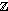21;

• (b)  the multiplicative inverse of 19 in33.

Solution

• (a)

•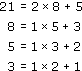• Hence

•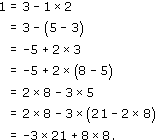• Hence 8 × 8 = 3 × 21 + 1, so

•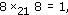• so the multiplicative inverse of 8 in21 is 8.

• (b)

•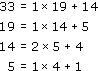• Hence

•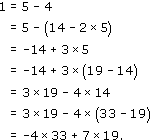• Hence

•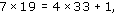• so

•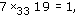• so the multiplicative inverse of 19 in33 is 7.

Exercise 53

Construct the multiplication table for11, and hence find the multiplicative inverse of every non-zero element in11.

Solution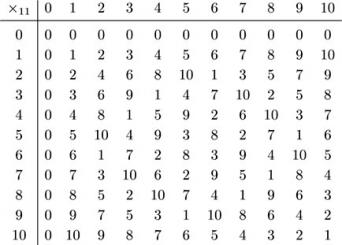Hence we have the following multiplicative inverses in11.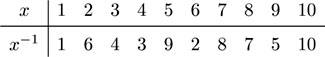Exercise 54

Use the solution to Exercise 52 to solve the following equations.

• (a)  8 ×21x = 13

• (b)  19 ×33x = 15

Solution

• (a)  We have 8 ×21x = 13. Multiplying by 8, which is the multiplicative inverse of 8 mod 21 (see the solution to Exercise 52(a)), we have

•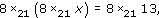• so

•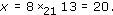• (b)  We have 19 ×33x = 15. Multiplying by 7, which is the multiplicative inverse of 19 mod 33 (see the solution to Exercise 52(b)), we have

•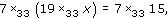• so

•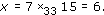Exercise 55

Find all the solutions of the following equations.

• (a)  In8: 3 ×8x = 7,      4 ×8x = 7,    4 ×8x = 4.

• (b)  In15: 3 ×15x = 6,    4 ×15x = 3,    5 ×15x = 2.

Solution

• (a)  Because 3 and 8 are coprime, the equation 3 ×8x = 7 has a unique solution. The solution, x = 5, can be found in various ways: for example, by calculating x = 3−1 ×8 7, or by testing possible values for x, or by spotting that 7 ≡ 15 (mod 8) and using the fact that the congruence 3 × 5 ≡ 15 (mod 8) implies 3 × 5 ≡ 7 (mod 8) giving 3 ×8 5 = 7.

• The equation 4 ×8x = 7 has no solutions because 4 and 8 have common factor 4 but 7 does not.

• One solution of the equation 4 ×8x = 4 is x = 1. Also n/d = 8/4 = 2, so the other solutions are x = 1 + 2 = 3, x = 1 + 4 = 5 and x = 1 + 6 = 7.

• (b)  One solution of the equation 3 ×15x = 6 is x = 2. Also n/d = 15/3 = 5, so the other solutions are x = 2 + 5 = 7 and x = 2 + 10 = 12.

• Because 4 and 15 are coprime, the equation 4 ×15x = 3 has a unique solution. The solution, x = 12, can be found in various ways: for example, by calculating x = 4−1 ×15 3, or by testing possible values for x, or by spotting that 3 ≡ −12 (mod 15) and using the fact that the congruence 4 × (−3) ≡ −12 (mod 15) implies 4 × 12 ≡ 3 (mod 15) giving 4 ×15 12 = 3.

• The equation 5 ×15x = 2 has no solutions because 5 and 15 have common factor 5 but 2 does not.

Exercise 56

• (a)  Show that the equation x ×12x = 7 has no solutions.

• (b)  Find all the solutions of x ×12x = 4.

Solution

We find all the values of x ×12x.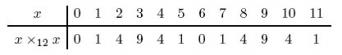• (a)  Hence there is no integer x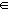12 such that x ×12x = 7.

• (b)  The solutions of x ×12x = 4 are x = 2, 4, 8, 10.

M208_6